Measuring the Torque Constant kT

﻿

SPS / IPC / Drives 2005: Indirekte Messung der winkelabhängigen Drehmomentkonstante kT für Direktantriebe
English version is translated from German original version.

Introduction

In automation technology, a machine's throughput as well as the quality of a product essentially depend on the motion technology. Often, you can considerably increase both a machine's throughput and the product quality by using direct drives. To achieve the desired dynamics, in case of the used control loops, often the speed control as well as the torque control are precontrolled (cf. picture 1). In case of the mostly used permanently excited synchronous motors, the torque is controlled indirect by setting the current component iq(t) which creates the torque. In case of motors without cogging, and with an ideal induced sinusoidal tension (EMF), this does not cause any problems (1).

Cogging can be minimized by a good motor design combined with a precise fabrication. If "ironless" motors are used (motors that do not contain any iron in the area of the winding), principally there cannot occur any cogging torque. There are no motors with principal sinusoidal EMF. Especially in case of high pole direct drives with bearing in the machine, it is hardly possible to precisely observe the air gap. In case of  ironless motors, the magnetic flow is not guided by absiolute precisely manufactured lamination. Here, the course of the EMF is determined by the location of every winding.

Thus, it is desirable to be able to determine the EMFs with these motors installed in the machines. This is contradicted by the fact that machines are not similar to classic testing stands. Often, the speed is limited. Mostly, the drives cannot be operated at a constant speed as long as required. Thus, direct measurings of the induced tensions are usually not possible.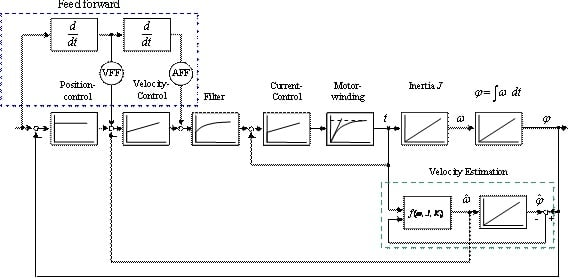Picture 1:  For the  highly dynamical control of a direct drive, you need  both a Velocity Feed Forward - VFF, and an Acceleration Feed Forward – AFF

Consideration of the fundamental physical equations

Disregarding the power dissipations,  in case of rotating machines, a motor's mechanical power is the product from its speed and torque,

pm(t) = m(t) * ω(t)                                               (1a)

or (in case of linear motors, it is the product of speed and force,
pm(t)  = F(t) · v(t)                                                 (1b)

The electrical power of permanently excited motors is calculated by the sum of the three separate phase powers:
pe(t) = uu(t) · iu(t) + uv(t) · iv(t) + uw(t) · iw(t)     (2)

As in case of  not connected neutral line neither the three tensions, nor the three currents are independent from each other,
iv(t) = –iu(t) – iw(t)

in order to offer more clarity, it  is better to choose spave vector design (Park & Clarke Transformation {2}) with only two tensions (ua and ub) and two currents (ia and ib) in rectangular coordinates:
pe(t) = ua · ia                                                       (3)
with
ia = iu – 0,5 ( iv + iw )                                        (4a)
and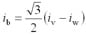(4b)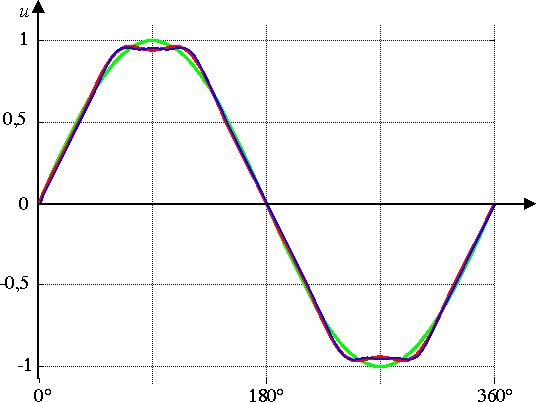Picture 2: induced tension  ui of one phase
Green:   sin(φ)
Red:     sin(φ) – 1/5² · sin(5·φ) + 1/7² · sin(7·φ)
Blue:   sin(φ) – 1/5² · sin(5·φ) + 1/7² · sin(7·φ) – 1/11² · sin(11·φ) + 1/13² · sin(13·φ)

In case of a sinusoidally induced tension (cf. picture 2), the components ua and ub of a three -phase system form an exact circle (cf. picture 3):
ua = û · cos( ω · t )                                                   (5a)
ub = û · sin( ω · t )                                                    (5b)

If also the current is impressed sinusoidally with the same phase, the electrical power is independent from the time t :
pe(t) = û · î · ( sin2( ω · t ) + cos2( ω · t ) ) = û · î    (6)

This consideration becomes much clearer if you recall the physical context of a permanently excited synchronous machine with  û ~ ω   and î ~ m .

However, if the induced tension is not exactly sinusoidal, the tension's space vector is not circular any more. Thus, the power, respectively the torque is not constant any more.

In case of the electronically commuted DC machines (Brushless DC, Six Step), the induced tension principally contains harmonic oscillations (cf. picture 3, blue curve).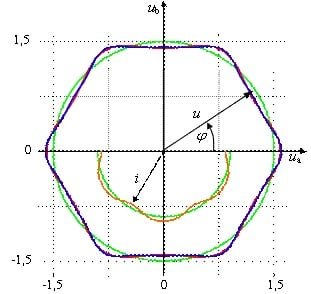Picture 3: induced tensions  ui (space vector)
Green:   sin(φ) without harmonic oscillations
Red:      sin(φ) + 5. aqnd 7.
Blue:      sin(φ) with 5. , 7. , 11., and 13.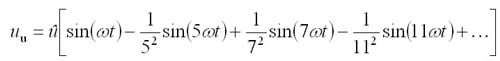Then, the tension space vector  follows a hexagon. It is easily visible that in the places or angles φ (t) = φ · t , where the EMF is especially small (e.g. φ = 30°), the tension must be correspondingly higher so that both  the momentary power p(t) or p(φ), and the torque are constant and therefore independent from the angle φ  .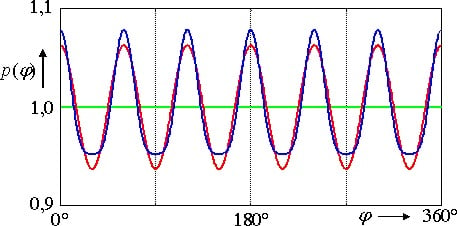Picture 4: momentary power p(j) of a servo motor in case of sinusoidal current
Green:    ui = sin(φ)
Red:      ui = sin(φ) – 1/5² · sin(5·φ) + 1/7² · sin(7·φ)
Blue:    ui = sin(φ) – 1/5² · sin(5·φ) + 1/7² · sin(7·φ) – 1/11² · sin(11·φ) + 1/13² · sin(13·φ)

Picture  4 (blue) shows the course of the momentary power p(φ) of a brushless DC motor at constant speed operated with a constant sinusoidal current.
In case of both a constant current (in rotor coordinates) and a constant speed - this course corresponds to the course of the induced tension uq in rotor coordinates.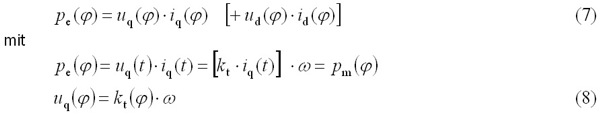In case of synchronous machines without weakening of field, the component ud(t) influences neither the torque nor the power. As at a constant speed the power is not constant, consequently, the torque can neither be constant.
The context m = kt · iq can only describe this reaction, because kt is considered to be the „ angle dependent torque constant “ kt(φ).
As the course of kt(φ) depends on the angle, but not on the torque, in the relation pe(t) = pm(t) the condition of the constant speed  becomes insifignicant.
In case of an ideal brushless DC motor, kt(φ)  would take the following course: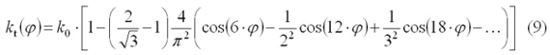If such a motor is fed with sinusoidal current, the torque can be controlled very precise, if the current set point  is weighted dependent from the angle(φ) by means of a function reciprocal to kt(φ) (see picture 2).
Now it is of special interest, how harmonic oscillations of the induced tension measured in stator coordinates affect kt(φ) :
Only the fundamental oszillation a1 · sin(ω t) corresponds to the desired course. It corresponds to a constant in rotor coordinates.
The undesirable harmonic oscillations
an · sin(n·ω t) + bn · cos (n·ω t)                                            (10)
can be subdivided into different categories:

• Theoretically, cos( )-terms do not arise from balance considerations (bi = 0).
• Theoretically, harmonic oscillations with even multiples of fundamental oscillations do not arise from balance considerations  (a2 = 0, a4 = 0 , ... )
• Within a three - phase system (without center line), all harmonic oscillations that can be divided by three compensate themselves during a transformation to space vectors (Clarke)

Due to the construction, thus, only a small amount of the harmonic oscillations affects the course of the induced tensions in rotor coordinates:
a5 , a7 , a11 , a13 , a17 , a19 , ...

The course of the induced tension in field or rotor coordinates  (ω·kt(φ)) can be described by the following Fourier series:
uq(t) = c0 + c1 · cos(ω · t) + c2 · cos(2 · ω · t) + c3 · cos(3 · ω · t) + ...             (11a)
ud(t) = d0 + d1 · sin(ω · t) + d2 · sin(2 · ω · t) + d3 · sin(3 · ω · t) + ...               (11b)

At the transformation in rotor coordinates, there result the following relations:

 c0 = a1 d0 = 0 (=b1) (12) c3 = a4 – a2 d3 = a2 + a4 c6 = a7 – a5 d6 = a5 + a7 c9 = a10 – a8 d9 = a8 + a10 c12 = a13 – a11 d12 = a11 + a13 c15 = . . .

This behavior can be summarized as follows:

• A course of the induced tension in stator coordinates (a5 , a7 , a11 , a13 , a17 , a19 , ...), which, due to construction, is not sinusoidal, influences the harmonic oscillations that can be divided by six (c6 , c12 , c18 , ...) of the torque constant kt(φ) (~ induced tension in rotor coordinates).
• All other possibly measured coefficients or factors hint to mechanical or electrical unsymmetries of the motor.
• With only the measured induced tension in rotor coordinates (uq), you cannot completely calculate the course in stator coordinates (ud is missing).
• For example, for a BLDC motor the results are c6 = 0,06 and c12 = 0,014 .

Measuring the induced tension on the testing stand

In case of industrially manufactured motors, it is easy to measure the induced tension. With a simple op amp circuit, the  motor's three - phase induced tension can be transformed into space vector signals. Picture 5 shows the course of the tension which is neither circular nor exactly hexagonal.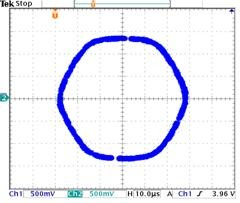Picture 5 : measured course of the induced tension in space vector representation

Measuring kt(φ) on the testing stand

Another possibility is to measure kt(φ) at very low speed. On a testing stand, the exact measuring of currents and torques doesn't cause any problems. However, using this method of measuring, you must not disregard disturbance variables like friction or cogging.
Therefore, in case of conventional motors, kt(φ) is mostly established by measuring the induced tension.

Measuring kt(φ) inside the machine

In many applications with direct drives, it is not possible to measure kt(φ) inside the machine, as you can neither operate the machine at a constant speed (or velocity), neither exactly measure the torque (or the force) without using additional devices. However, just in case of these applications an exact measurement of kt(φ) would be desirable:

• In case of direct drives, it is not always guaranteed that the orientation of the coil and the magnetic track or the air gap corresponds to the specifications.
• Often, direct drives are chosen because the machine shall possess a maximum of dynamics and precision.

If the moment of inertia J, (or the mass m) are constant, the torque m (or the force F) can be determined indirectly by measuring the acceleration a :

F = m · a                                     (13)

In the case of highly dynamical drives, exact measurement of the acceleration is not unproblematic as well. The easier and significantly more exact way is to measure the loop gain of the cur speed control loop (open loop) at a  given constant loop frequency ω0 as function of the position {3}.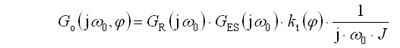(14)

In a solid radiant frequency ω0,  the transfer functions

• of the speed control GR(jω0),
• of the closed current control loop GES(jω0) and
• of the weight  1 / (j · ω0 · J )

are constant. The consequence of this is that with a constant moment of inertia J, the loop gain of the open speed control loop is directly proportional to the torque constant:

G0 (jω0,φ) ~ kt (φ)

Picture 6 shows the course of the loop gain as a function of the mechanical angle G0 (φ) .
In picture 7 the measured course of the loop gain (induced tension) is applied. The course corresponds to the directly measured course as represented in picture 5.
Picture 8 shows the coefficients or factors ci of the induced tension after a Fourier analysis. These data were measures with a S300 and the ASCII command.
„VKT 100 360 360“
Parameter: 100 Hz (ω0 = 628 s-1) over  360 degrees with  360 measuring points {4}.

If, while measuring G0 (jω0,φ), the DC constant is also measured,  from the resulting course you can deduce the cogging.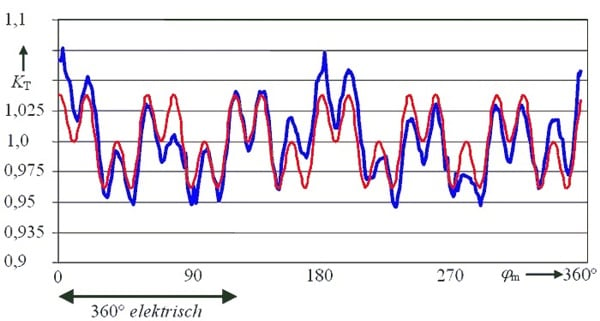Picture 6: indirectly measured course of the induced tension
blue: K T as function of the mechanical angle (6-pole motor)
red: convergence due to the  6th and the 18th harmonic oscillation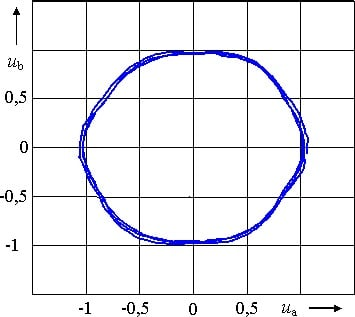Picture 7: indirectly measured course of the induced tension in the space vector representation

Summary

Especially in case of direct drives without motor - sided bearing it is not unproblematic to exactly keep the air gap. This can result in undesired cogging torque, and a not exactly sinusoidally induced tension (EMF). In the case of direct drives that have already been installed in machines, and that usually turn slowly and often only rotate less than one turn, it is hardly possible to measure the induced tension without using additional tools. With the new procedure, the servo amplifier can measure the form of the tension very exactly directly in the machine without using further devices. Taking these data as bases, it is possible now to very precisely effect the respective torque's feed forward that mostly is required in direct drives .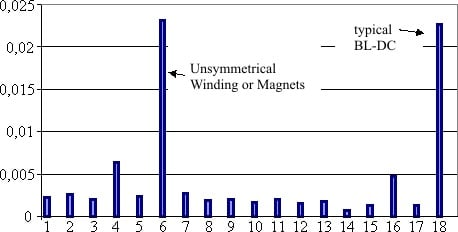Picture 8: Fourier's coefficient of the indirectly measured force constant KT(jm)
The  18th of a "mechanical turn“ corresponds to the 6th of an "electrical turn"

The essay shows how to exactly measure the form of the induced tension with a "normal" drive, consisting of a servo amplifier and a motor. Based on the deviation from the ideal sine form, you can determine the angle - dependant torque constant  kt(φ). With this angle - dependant factor the torque can be precontrolled in a significantly more exact way.
This procedure is demonstrated using a motor with trapezoidal (Brushless DC) EMF. For this purpose, firstly, the EMF is measured directly at constant speed, and then it is compared with the result of the new indirect measurement.

Bibliography

1. Röhrig, Christof: Zur Lageregelung synchroner Linearmotoren für hochdynamische Anwendungen unter besonderer Berücksichtigung der Kraftwelligkeit, Dissertation - Fortschritt Berichte VDI, Düsseldorf 2003.
2. L. Springob, J. Holtz: High Bandwidth Current Control for Torque Ripple Compensation in PM Synchronous Machines. IEEE Transactions on Industrial Electronics, Vol. 45, No. 5, 1998, pp. 713-721.
3. J. O. Krah: Bode Plot based Autotuning – Enhanced Solution for High Performance Servo Drives. PCIM Power Conversion Intelligent Motion, Nürnberg, May 2004, pp. 382-387 .
4. Manuals S300

Author

Prof. Dr.-Ing. Jens Onno Krah
Fachhochschule Köln
Fakultät IME – NT